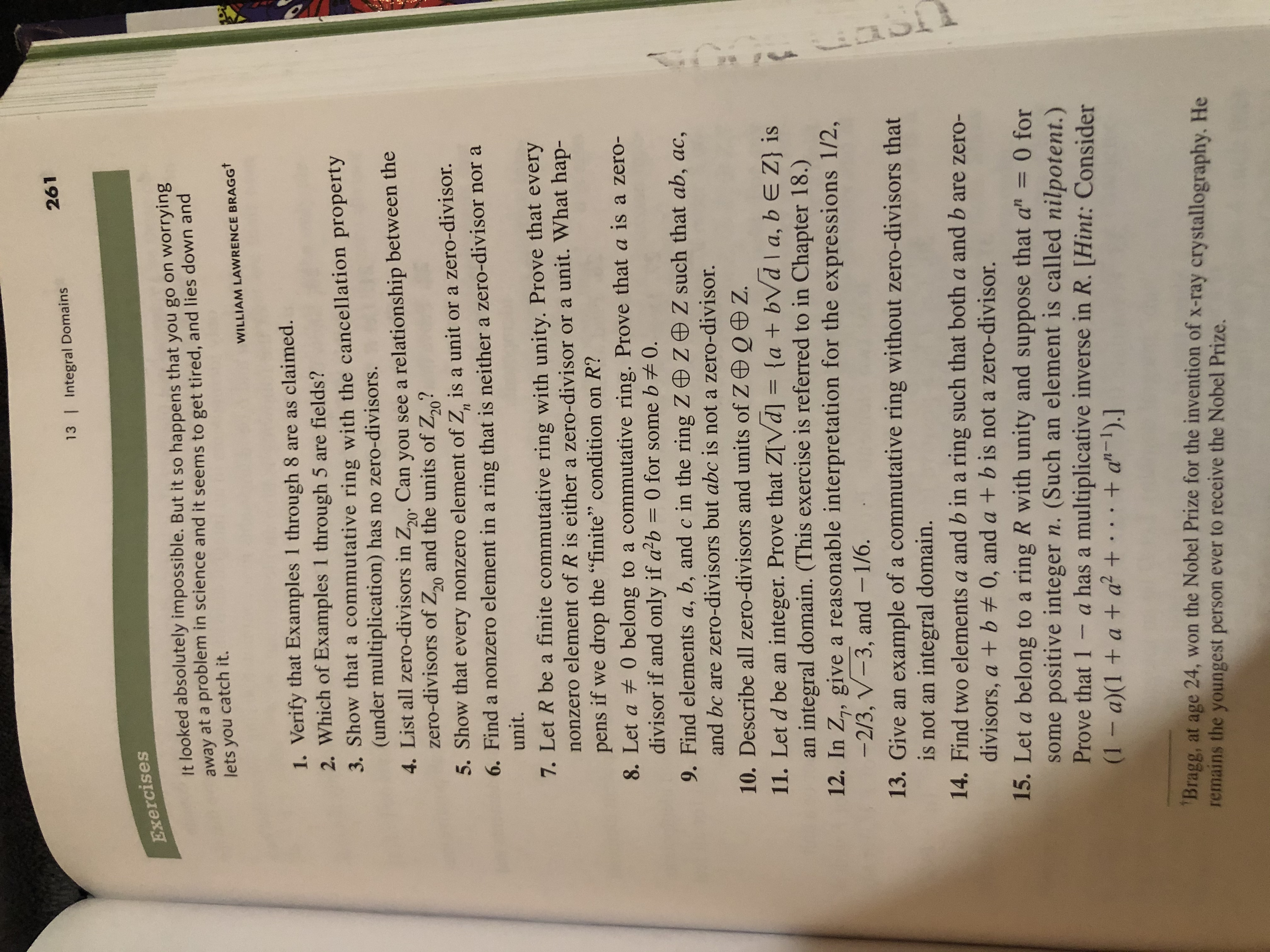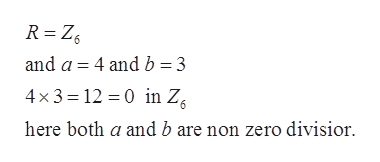# 26113 | Integral DomainsExercisesIt looked absolutely impossible. But it so happens that you go on worryin8at a problem in science and it seems to get tired, and lies down andawaylets you catch it.WILLIAM LAWRENCE BRAGGT1. Verify that Examples 1 through 8 are as claimed.2. Which of Examples 1 through 5 are fields?3. Show that a commutative ring with the cancellation property(under multiplication) has no zero-divisors.4. List all zero-divisors in Z2o: Can you see a relationship between thezero-divisors of Z and the units of Z20?205. Show that every nonzero element of Z is a unit or a zero-divisor.6. Find a nonzero element in a ring that is neither a zero-divisor nor aunit.п7. Let R be a finite commutative ring with unity. Prove that everynonzero element of R is either a zero-divisor or a unit. What hap-pens if we drop the "finite" condition on R?8. Let a 0 belong to a commutative ring. Prove that a is a zero-divisor if and only if a2b 0 for some b 0.9. Find elements a, b, and c in the ring Z ZeZ such that ab, ac,and bc are zero-divisors but abc is not a zero-divisor.10. Describe all zero-divisors and units of ZOQZ11. Let d be an integer. Prove that Z[Vd] = {a + bVd a, b E Z} isan integral domain. (This exercise is referred to in Chapter 18.)12. In Z,, give a reasonable interpretation for the expressions 1/2,-2/3, V-3, and - 1/6.13. Give an example of a commutative ring without zero-divisors thatis not an integral domain.14. Find two elements a and b in a ring such that both a and b are zero-Cpadivisors, a + b 0, and a + b is not a zero-divisor.15. Let a belong to a ring R with unity and suppose that a" = 0 forsome positive integer n. (Such an element is called nilpotent.)Prove that 1 a has a multiplicative inverse in R. [Hint: Consider(1- a)(1 + a + a2+ + a-1).]Bragg, at age 24, won the Nobel Prize for the invention of x-ray crystallography. Heremains the youngest person ever to receive the Nobel Prize.

Question
4 views

14help_outlineImage Transcriptionclose261 13 | Integral Domains Exercises It looked absolutely impossible. But it so happens that you go on worryin8 at a problem in science and it seems to get tired, and lies down and away lets you catch it. WILLIAM LAWRENCE BRAGGT 1. Verify that Examples 1 through 8 are as claimed. 2. Which of Examples 1 through 5 are fields? 3. Show that a commutative ring with the cancellation property (under multiplication) has no zero-divisors. 4. List all zero-divisors in Z2o: Can you see a relationship between the zero-divisors of Z and the units of Z20? 20 5. Show that every nonzero element of Z is a unit or a zero-divisor. 6. Find a nonzero element in a ring that is neither a zero-divisor nor a unit. п 7. Let R be a finite commutative ring with unity. Prove that every nonzero element of R is either a zero-divisor or a unit. What hap- pens if we drop the "finite" condition on R? 8. Let a 0 belong to a commutative ring. Prove that a is a zero- divisor if and only if a2b 0 for some b 0. 9. Find elements a, b, and c in the ring Z ZeZ such that ab, ac, and bc are zero-divisors but abc is not a zero-divisor. 10. Describe all zero-divisors and units of ZOQZ 11. Let d be an integer. Prove that Z[Vd] = {a + bVd a, b E Z} is an integral domain. (This exercise is referred to in Chapter 18.) 12. In Z,, give a reasonable interpretation for the expressions 1/2, -2/3, V-3, and - 1/6. 13. Give an example of a commutative ring without zero-divisors that is not an integral domain. 14. Find two elements a and b in a ring such that both a and b are zero- C pa divisors, a + b 0, and a + b is not a zero-divisor. 15. Let a belong to a ring R with unity and suppose that a" = 0 for some positive integer n. (Such an element is called nilpotent.) Prove that 1 a has a multiplicative inverse in R. [Hint: Consider (1- a)(1 + a + a2+ + a-1).] Bragg, at age 24, won the Nobel Prize for the invention of x-ray crystallography. He remains the youngest person ever to receive the Nobel Prize. fullscreen
check_circle

Step 1

Zero-divisors - An element a of a ring R is called a zero divisor if there exists a nonzero x such that ax = 0.

Step 2

Zero-divisors - An element a of a ring R is called a zero divisor if there exists a nonzero x such that ax = 0.

Step 3

Let

...help_outlineImage TranscriptioncloseR Z6 and a 4 and b 3 4x3 12 0 in Z here both a and b are non zero divisior fullscreen

### Want to see the full answer?

See Solution

#### Want to see this answer and more?

Solutions are written by subject experts who are available 24/7. Questions are typically answered within 1 hour.*

See Solution
*Response times may vary by subject and question.
Tagged in

### Math